Paper :

The goal of this paper is to take a video and a task image as input and apply the task image on the entire video. This task could be pixel segmentation, pixel colorization, etc.

## Models

To achieve this they use two kinds of network, a Bilateral network, and a Spatial network.

The bilinear features used are defined by a 6 dimensional feature vector $$F^i = (x, y, Y, Cb, Cr, t)^T$$. Where $$x$$ and $$y$$ are the pixel position in the image, $$Y$$, $$Cb$$, and $$Cr$$ the luma, blue-difference and red-difference chroma components, and $$t$$ the time of the frame in the video.

The bilateral network performs video-adaptive spatiotemporal dense filtering that is given to the spatial network (regular CNN) used to produce the new segmentation from the previous frame segmentation as shown in Fig.3.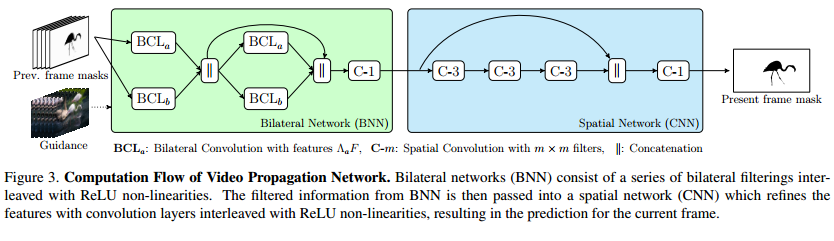This model is used in a staged flavor by first generating a segmentation and refining it recursively.

## Evaluation

The VPN model is tested on three different propagation task: foreground mask, semantic labeling and color propagation.

They use the DAVIS dataset to evaluate their model. The tables show three metrics for the evaluation, Intersection over Union (IoU), contour accuracy ($$\mathcal{F}$$) and the temporal instability score ($$\mathcal{T}$$).

• The results on Video Object Segmention of DAVIS: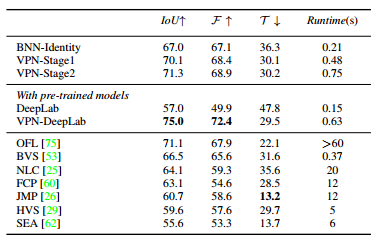Their model with pretraining achieves an improvement of 5% on all the metrics while being 10 times faster than the best reported method (OFL).

• The results on Semantic segmentation on CamVid dataset: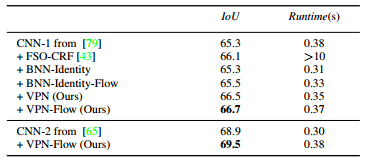• The results on DAVIS for color propagation: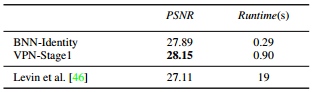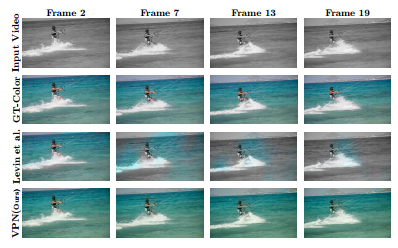They manage to improve the result in term of PSNR values and again being 20 time faster than the reported model.